NCERT Solutions for Class 7 Maths Chapter 5 Lines and Angles Ex 5.1 are part of NCERT Solutions for Class 7 Maths. Here we have given NCERT Solutions for Class 7 Maths Chapter 5 Lines and Angles Ex 5.1.

 Board CBSE Textbook NCERT Class Class 7 Subject Maths Chapter Chapter 5 Chapter Name Lines and Angles Exercise Ex 5.1, Ex 5.2. Number of Questions Solved 14 Category NCERT Solutions

## NCERT Solutions for Class 7 Maths Chapter 5 Lines and Angles Ex 5.1

Question 1.
Find the complement of each of the following angles:Solution:
Since, the sum of the measures of an angle and its complement is 90°, therefore,

1. The complement of an angle of measure 20° is the angle of (90° – 20°), f.e., 70°.
2. The complement of an angle of measure 63° is the angle of (90° – 63°), i.e., 27°.
3. The complement of an angle of measure 57° is the angle of (90° – 57°), i.e., 33°.

Question 2.
Find the supplement of each of the following angles:Solution:

1. Supplement of the angle 105° = 180° – 105° = 75°
2. Supplement of the angle 87° = 180° – 87° = 93°
3. Supplement of the angle 154° = 180° – 154° = 26°

Question 3.
Identify which of the following pairs of angles are complementary and which are supplementary.

1. 65°, 115°
2. 63°, 27°
3. 112°, 68°
4. 130°, 50°
5. 45°,45°
6. 80°, 10°.

Solution:

1. Since, 65°+ 115° = 180°
So, this pair of angles are supplementary.
2. Since, 63°+ 27° = 90°
So, this pair of angles are complementary.
3. Since, 112° + 68° = 180°
So, this pair of angles are supplementary.
4. Since, 130°+50° = 180°
So, this pair of angles are supplementary.
5. Since, 45°+ 45° = 90°
So, this pair of angles are complementary.
6. Since, 80°+ 10° = 90°
So, this pair of angles are complementary.

Question 4.
Find the angle which is equal to its complement.
Solution:
Let the measure of the angle be x°. Then, the measure of its complement is given to be x°.
Since, the sum of the measures of an angle and its complement is 90°, therefore,
x° + x° = 90°
⇒ 2x° = 90°
⇒ x° = 45°
Thus, the required angle is 45°.

Question 5.
Find the angle which is equal to its supplement.
Solution:
Let the measure of the angle be x°. Then,
a measure of its supplement = x°
Since, the sum of the measures of an angle and its supplement is 180°, therefore,
x° + x° = 180°
⇒ 2x° =180°
⇒ x° = 90°
Hence, the required angle is 90°.

Question 6.
In the given figure, ∠ 1 and ∠ 2 are supplementary angles.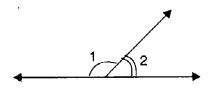If ∠1 is decreased, what changes should take place in ∠ 2 so that both the angles still remain supplementary?
Solution:
∠ 2 will increase as much as ∠ 1 decreases.

Question 7.
Can two angles be supplementary if both of them are:

1. acute?
2. obtuse?
3. right?

Solution:

1. No! two acute angles cannot be a supplement.
2. No! Two obtuse angles cannot be supplementary.
3. Yes! Two right angles are always supplementary.

Question 8.
An angle is greater than 45°. Is its complementary angle greater than 45° or equal to 45° or less than 45°.
Solution:
Since the sum of the measure of ah angle and its complement is 90°.
∴ The complement of an angle of measures 45° + x°,
where x > 0 is the angle of [90° – (45° + x°)] = 90° – 45° – x°= 45° – x°.
Clearly, 45° + x° > 45° – x°
Hence, the complement of an angle > 45° is less than 45°.

Question 9.1. Is ∠1 adjacent to ∠2 ?
2. Is ∠ AOC adjacent to ∠ AOE?
3. Do ∠ COE and ∠ EOD form a linear pair?
4. Are ∠ BOD and ∠ DOA supplementary?
5. Is ∠ 1 vertically opposite to ∠ 4?
6. What is the vertically opposite angle of ∠ 5?

Solution:

1. Yes ! ∠ 1 is adjacent to ∠ 2.
2. No ! ∠ AOC is not adjacent to ∠ AOE.
3. Yes! ∠ COE and ∠ EOD form a linear pair.
4. Yes ! ∠ BOD and ∠ DOA are supplementary.
5. Yes ! ∠ 1 is vertically opposite to ∠ 4.
6. The vertically opposite angle of ∠ 5 is ∠ 2 + ∠ 3, i.e., ∠ COB.

Question 10.
Indicate which pairs of angles are: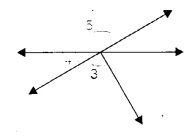1. Vertically opposite angles.
2. Linear pairs.

Solution:

1. The pair of vertically opposite angles are ∠1, ∠4; ∠5, ∠2 + ∠3.
2. The pair of linear angles are ∠1, ∠5; ∠4, ∠5.

Question 11.
In the following figure, is ∠ 1 adjacent to ∠ 2? Give reasons.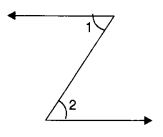Solution:
∠1 is not adjacent to ∠2 because they have no common vertex.

Question 12.
Find the values of the angles x, y, and z in each of the following: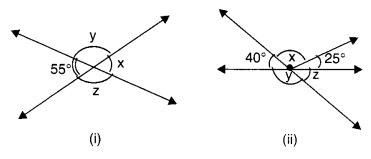Solution: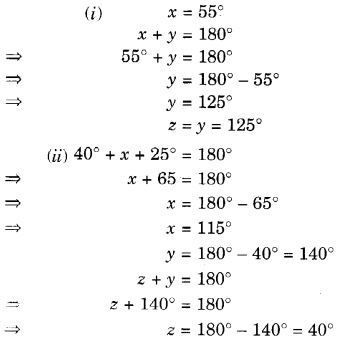Question 13.
Fill in the blanks:

1. If two angles are complementary, then the sum of their measures is
2. If two angles are supplementary, then the sum of their measures is
3. Two angles forming a linear pair are
4. If two adjacent angles are supplementary, they form a
5. If two lines intersect at a point, then the vertically opposite angles are always
6. If two lines intersect at a point, and if one pair of vertically opposite angles are acute angles, then the other pair of vertically opposite angles are

Solution:

1. 90°
2. 180°
3. supplementary
4. linear pair
5. equal
6. obtuse angles

Question 14.
In the adjoining figure, name the following pairs of angles.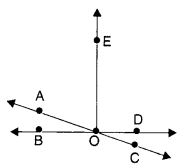1. Obtuse vertically opposite angles